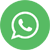EXPLORATORY DATA ANALYSIS IN DATA SCIENCE

## EXPLORATORY DATA ANALYSIS IN DATA SCIENCE

1.Understand the defined Business Objective

2.Research & explore on the Domain knowledge or consult Subject matter expert (SME)

3.Collect the metadata of the given data with the help of SME or explore various research avenues

4.Collect the data for the variables which are relevant for the project based on domain expertise

5.Data cleansing & wrangling to be performed to make data structured

# Dummy variable creation

a.Create Dummy variable for categorical data in binary format(1 or 0) if exists of two levels in a factor
b.If more than two levels in a factor create dummy column with each level
Imputation for missing data
There are many types of imputation techniques which replaces N/A values
a.List wise deletion (Complete Case Analysis) Delete whole row if any N/A found
b.Pair wise deletion (Available Case Analysis)Delete the particular cell or value
c.Mean imputation-Replaces the N/A Value with Mean of the Variable
d.Mode imputation-Replaces the N/A value with Mode of the Variable
e.Hot deck Imputation-Replaces the similar value by checking each row
f.Regression Imputation-N/A is considered as an output and replace it by predicting the value
g.KNN Imputation-By Calculating the distance between each data point and replaced with the nearest neighbour

6.Find out the data types (Continuous, Discrete, Nominal, Ordinal, Interval, Ratio)

7.Find the Probability of the data
No. of interested events / Total no. of events

8.Find the Data to which probability distribution it belongs to

Probability distribution will always have Random Variable on X-axis & Probabilities associated with random variables on Y-axis
a.Continuous Probability Distribution
b.Discrete Probability distribution

9.Find whether the data is following normal distribution
a.Symmetrical
b.Bell shaped curve
c.Mean = 0, area under the curve = 1

10.If data is not following normal distribution, then transform the data.

11.Various types of transformations:

a.Log transformation.
b.1/log
c.square
d.Square root
e.1/ square root
f.Exponential
g.Cube
h.Cube root
i.1/ cube root
j.Boxcox transformation
k.Johnson transformation, And many more Transformations ….

12.If despite transformation data follow normal distribution, then perform analysis pertaining to non-normal distribution

13.Standard normal distribution (Z Distribution)
(X-µ/sigma)
Mean = 0, Standard Deviation = 1

14.Measures of Central Tendency (or) 1st Moment Business Decision
a.Mean
Average of the particular variable (Xi/n)
b.Median
Middle most number
c.Mode
Most repeated value

15.Measures of Dispersion (or) 2nd Moment Business Decision
a.Variance
Var(X) = E[(X-µ) ^2]
Distance from mean to each point, where units gets squared
b.Standard Deviation
Sqrt of Variance, where units get normal (sqrt(var))
c.Range: Max(Xi) – Min(Xi)

16.Measures of Skewness (or) 3rd Moment Business decision
a.Positive Skewed (or) Right skewed
b.Negative Skewed (or) Left Skewed

17.Measures of Kurtosis (or) 4th Moment Business Decision
a.Positive Kurtosis (or) Thinner peak
b.Negative Kurtosis (or) Wider Peak

18.Graphical Representation
a.Histogram
Represents the Normal Distribution of data, Skewness
b.Boxplot
Represents the outliers, median, Q1, Q3.
c.Bar plot
Represents the Data
d.Stem and leaf plot
A Stem and Leaf Plot is a special table where each data value is split into a “stem” (the first digit or digits) and a “leaf” (usually the last digit)
E.g.: – 32 -> Stem ‘3’ Leaf ‘2’
e.Dot plot
Represents the normal distribution and skewness